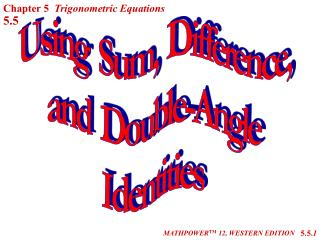# Using Sum, Difference, and Double-Angle Identities - PowerPoint PPT PresentationDownload PresentationUsing Sum, Difference, and Double-Angle Identities

Using Sum, Difference, and Double-Angle Identities
Download Presentation## Using Sum, Difference, and Double-Angle Identities

- - - - - - - - - - - - - - - - - - - - - - - - - - - E N D - - - - - - - - - - - - - - - - - - - - - - - - - - -
##### Presentation Transcript

1. Chapter 5 Trigonometric Equations 5.5 Using Sum, Difference, and Double-Angle Identities 5.5.1 MATHPOWERTM 12, WESTERN EDITION

2. Sum and Difference Identities sin(A + B) = sin A cos B + cos A sin B sin(A - B) = sin A cos B - cos A sin B cos(A + B) = cos A cos B - sin A sin B cos(A - B) = cos A cos B + sin A sin B 5.5.2

3. Simplifying Trigonometric Expressions Express cos 1000 cos 800 + sin 800 sin 1000 as a trig function of a single angle. 1. 2. as a single trig function. Express 5.5.3

4. Finding Exact Values 1. Find the exact value for sin 750. Think of the angle measures that produce exact values: 300, 450, and 600. Use the sum and difference identities. Which angles, used in combination of addition or subtraction, would give a result of 750? sin 750 = 5.5.4

5. Finding Exact Values 2. Find the exact value for cos 150. cos 150 = 3. Find the exact value for 5.5.5

6. Using the Sum and Difference Identities Prove 5.5.6

7. Using the Sum and Difference Identities A B x y r 5.5.8

8. Double-Angle Identities The identities for the sine and cosine of the sum of two numbers can be used, when the two numbers A and B are equal, to develop the identities for sin 2A and cos 2A. cos 2A = cos (A + A) sin 2A = sin (A + A) Identities for sin 2x and cos 2x: 5.5.9

9. Double-Angle Identities Express each in terms of a single trig function. a)2 sin 0.45 cos 0.45 b) cos2 5 - sin2 5 Find the value of cos 2x for x = 0.69. 5.5.10

10. Double-Angle Identities Verify the identity 5.5.11

11. Double-Angle Identities Verify the identity 5.5.12

12. Double-Angle Equations 2p p y = cos 2A 5.5.13

13. Double-Angle Equations y = sin 2A 5.5.14

14. Identities Prove 5.5.16

15. Applying Skills to Solve a Problem The horizontal distance that a soccer ball will travel, when kicked at an angle q, is given by , where d is the horizontal distance in metres, v0 is the initial velocity in metres per second, and g is the acceleration due to gravity, which is 9.81 m/s2. a)Rewrite the expression as a sine function. Use the identity sin 2A = 2sin A cos A: 5.5.17

16. Applying Skills to Solve a Problem [cont’d] b)Find the distance when the initial velocity is 20 m/s. From the graph, the maximum distance occurs when The maximum distance is The graph of sin q reaches its maximum when Sin 2q will reach a maximum when Distance Angle q 5.5.18

17. Assignment Suggested Questions: Pages 272-274 A 1-16, 25-35 odd B 17-24, 37-40, 43, 47, 52 5.5.19